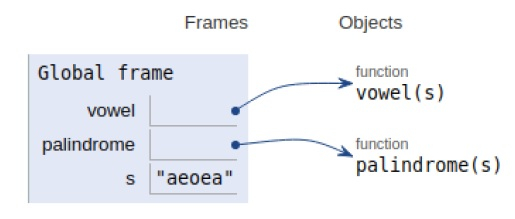# Python program to check if the given string is vowel Palindrome

PythonServer Side ProgrammingProgramming

#### Beyond Basic Programming - Intermediate Python

Most Popular

36 Lectures 3 hours

#### Practical Machine Learning using Python

Best Seller

91 Lectures 23.5 hours

#### Practical Data Science using Python

22 Lectures 6 hours

In this article, we will learn about the solution to the problem statement given below.

Problem statement − We are given string (containing both vowel and consonant letters), remove all consonants, then check if the resulting string is a palindrome or not.

Here we first remove all the consonants present in the string. A loop to calculate the divisors by computed by dividing each value from 1 to the minimum computed

Each time the condition is evaluated to be true counter is incremented by one.

Remove all the consonants in the string. Now we check whether the vowel string is a palindrome or not i.e. the given string and its reversal are identical or not. If it is a palindrome print YES, otherwise print NO. If the string contains no vowels(i.e. Only consonants), display -1.

Now let’s observe the concept in the implementation below−

## Example

Live Demo

def vowel(s):
flag=1
for c in s:
if c in "aeiou":
flag==1
else:
flag=0
break
if (flag==1):
return True
else:
return False
def palindrome(s):
if s==s[::-1]:
return True
else:
return False
# Driver Code
s = "aeoea"
if vowel(s) and palindrome(s):
print("It is a string palindrome")
else:
print("It is not a string palindrome")

## Output

It is a string palindromeAll the variables and functions are declared in the local scope and their references are seen in the figure above.

## Conclusion

In this article, we have learned about the python program to check whether a given string is a vowel palindrome or not.